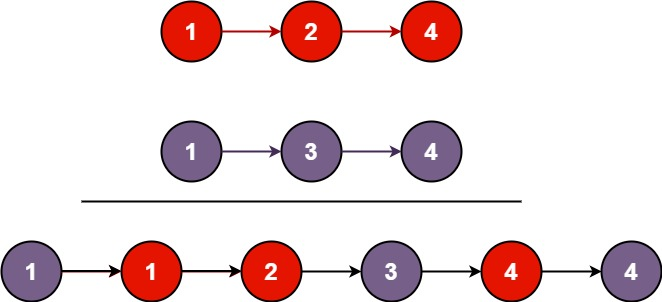# Amazon Online Assessment (OA) - Merge Two Sorted Lists

Merge two sorted linked lists and return it as a new sorted list. The new list should be made by splicing together the nodes of the given two lists.

## Examples:

### Example1:#### Input: `l1 = [1,2,4]`, `l2 = [1,3,4]`

Output: `[1, 1, 2, 3, 4, 4]`

### Example 2:

#### Input: `l1 = []`, `l2 = []`

Output: `[]`

### Example 3:

#### Input: `l1 = []`, `l2 = `

Output: ``

## Constraints:

The number of nodes in both lists is in the range `[0, 50]`.

• `-100 <= Node.val <= 100`
• Both `l1` and `l2` are sorted in non-decreasing order.

## Solution

 `Expand 3 lines ...` `4` `4` `` self.next = next`` `5` `5` `6` `6` ``def merge(l1: Node, l2: Node) -> Node:`` `7` `-` `` # WRITE YOUR BRILLIANT CODE HERE`` `7` `+` `` cur = dummy = Node(0)`` `8` `-` `` return None`` `8` `+` `` while l1 and l2:`` `9` `+` `` if l1.val < l2.val:`` `10` `+` `` cur.next, l1 = l1, l1.next`` `11` `+` `` else:`` `12` `+` `` cur.next, l2 = l2, l2.next`` `13` `+` `` cur = cur.next`` `14` `+` `15` `+` `` cur.next = l1 or l2`` `16` `+` `` return dummy.next`` `17` `+` `9` `18` ``def build_list(nodes, f):`` `10` `19` `` val = next(nodes, None)`` `11` `20` `` if val is None: return None`` `12` `21` `` nxt = build_list(nodes, f)`` `13` `22` `` return Node(f(val), nxt)`` `Expand 11 lines ...`